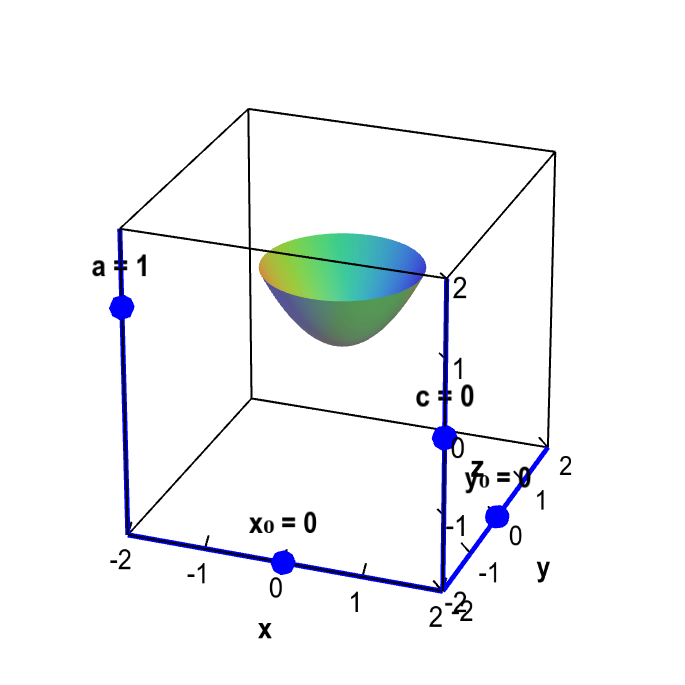# Math Insight

### Applet: Translation, rescaling, and reflectionYou can translate, rescale, and reflect of the graph $f(x,y)=x^2+y^2$, resulting in the graph of $z=af(x-x_0,y-y_0)+c$. Drag the sliders with your mouse to change $a$, $c$, $x_0$, and $y_0$. Changing $c$, $x_0$, and $y_0$ translates the graph. Changing the magnitude of $a$ rescales the graph; changing the sign of $a$ reflects the graph across the plane $z=c$.